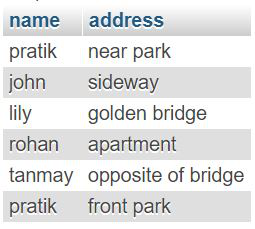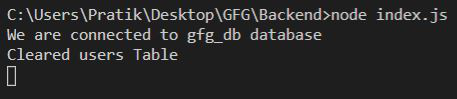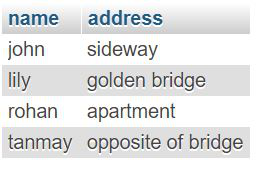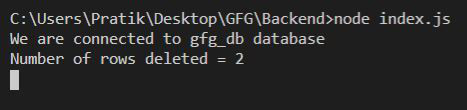# Node.js MySQL Delete Query

• Difficulty Level : Medium
• Last Updated : 07 Oct, 2021

We use SQL DELETE Query to delete data with some condition from MySQL Table.

Syntax:

• This will delete all rows of customers’ tables but everything else will remain as it is (indexing, etc).
`DELETE FROM users`
• This will delete all rows of users table where userId is 2.
`DELETE FROM users WHERE userId = 2`

Modules:

• mysql: To handle sql connection and queries
`npm install mysql`

SQL users table preview:Example 1: Delete all rows from users table

## Javascript

 `const mysql = require(``"mysql"``);`` ` `let db_con  = mysql.createConnection({``    ``host: ``"localhost"``,``    ``user: ``"root"``,``    ``password: ``''``,``    ``database: ``'gfg_db'``});`` ` `db_con.connect((err) => {``    ``if` `(err) {``      ``console.log(``"Database Connection Failed !!!"``, err);``      ``return``;``    ``}`` ` `    ``console.log(``"We are connected to gfg_db database"``);`` ` `    ``// here is our query``    ``let query = ``'DELETE FROM users'``;`` ` `    ``db_con.query(query, (err, rows) => {``        ``if``(err) ``throw` `err;`` ` `        ``console.log(``'Cleared users Table'``);``    ``});``});`

Output:Example 2: Delete all users whose name = ‘pratik’.

• Approach 1: Hard code the value

## Javascript

 `const mysql = require(``"mysql"``);`` ` `let db_con  = mysql.createConnection({``    ``host: ``"localhost"``,``    ``user: ``"root"``,``    ``password: ``''``,``    ``database: ``'gfg_db'``});`` ` `db_con.connect((err) => {``    ``if` `(err) {``      ``console.log(``"Database Connection Failed !!!"``, err);``      ``return``;``    ``}`` ` `    ``console.log(``"We are connected to gfg_db database"``);`` ` `    ``// This is our Hard Coded Query``    ``let query = ``"DELETE FROM users WHERE name = 'pratik'"``;`` ` `    ``db_con.query(query, (err, rows) => {``        ``if``(err) ``throw` `err;`` ` `        ``console.log(``'Number of rows deleted = '` `+ rows.affectedRows);``    ``});``});`
• Approach 2: Dynamic Query

## Javascript

 `const mysql = require(``"mysql"``);`` ` `let db_con  = mysql.createConnection({``    ``host: ``"localhost"``,``    ``user: ``"root"``,``    ``password: ``''``,``    ``database: ``'gfg_db'``});`` ` `db_con.connect((err) => {``    ``if` `(err) {``      ``console.log(``"Database Connection Failed !!!"``, err);``      ``return``;``    ``}`` ` `    ``console.log(``"We are connected to gfg_db database"``);`` ` `    ``// notice the ? in below query``    ``let query = ``"DELETE FROM users WHERE name = ?"``;``     ` `    ``// Dynamic Value``    ``let name = ``'pratik'``;`` ` `    ``// Notice the name variable below``    ``// It will replace the ? in query``    ``db_con.query(query, name, (err, rows) => {``        ``if``(err) ``throw` `err;`` ` `        ``console.log(``'Number of rows deleted = '` `+ rows.affectedRows);``    ``});``});`

Output:My Personal Notes arrow_drop_up